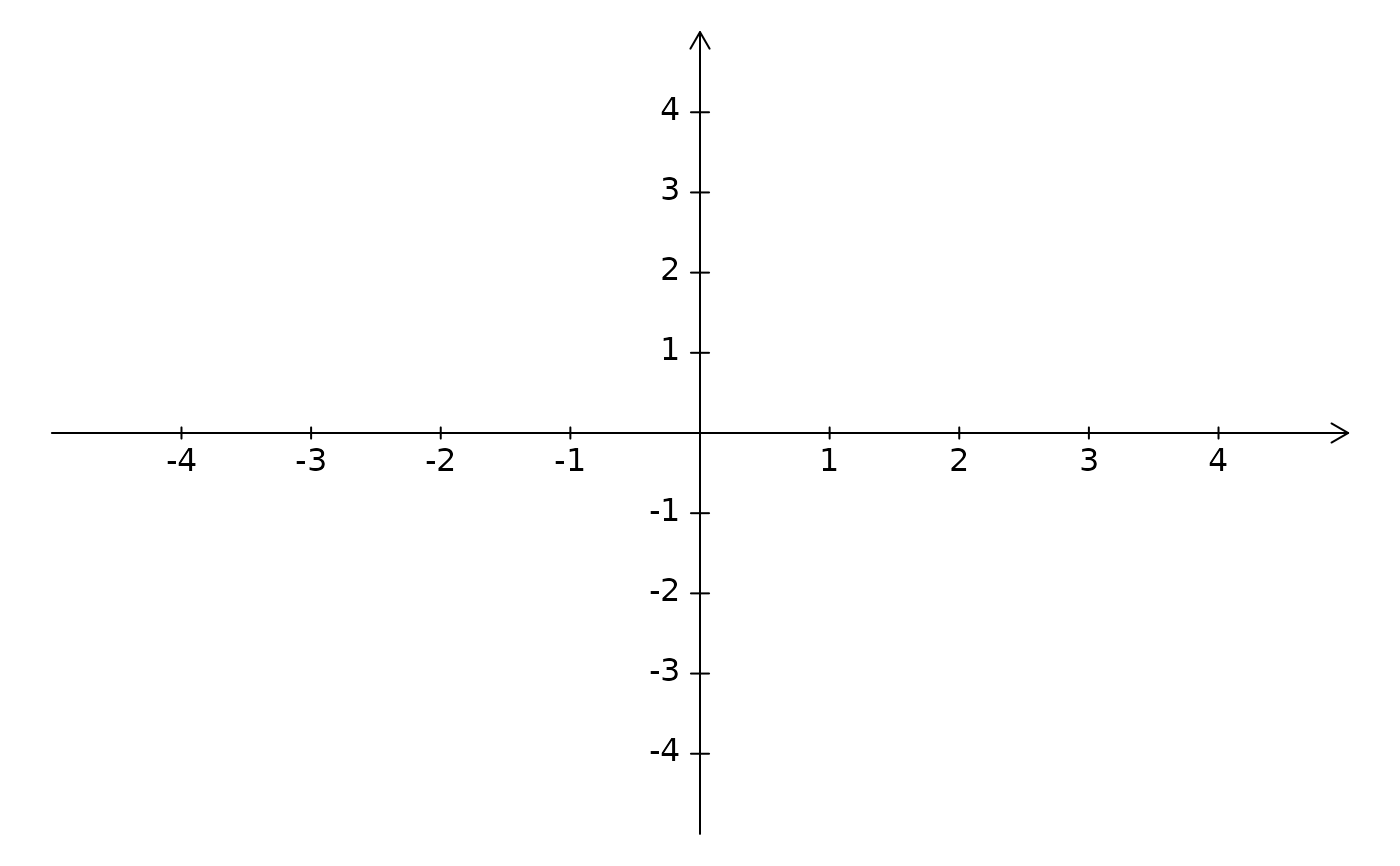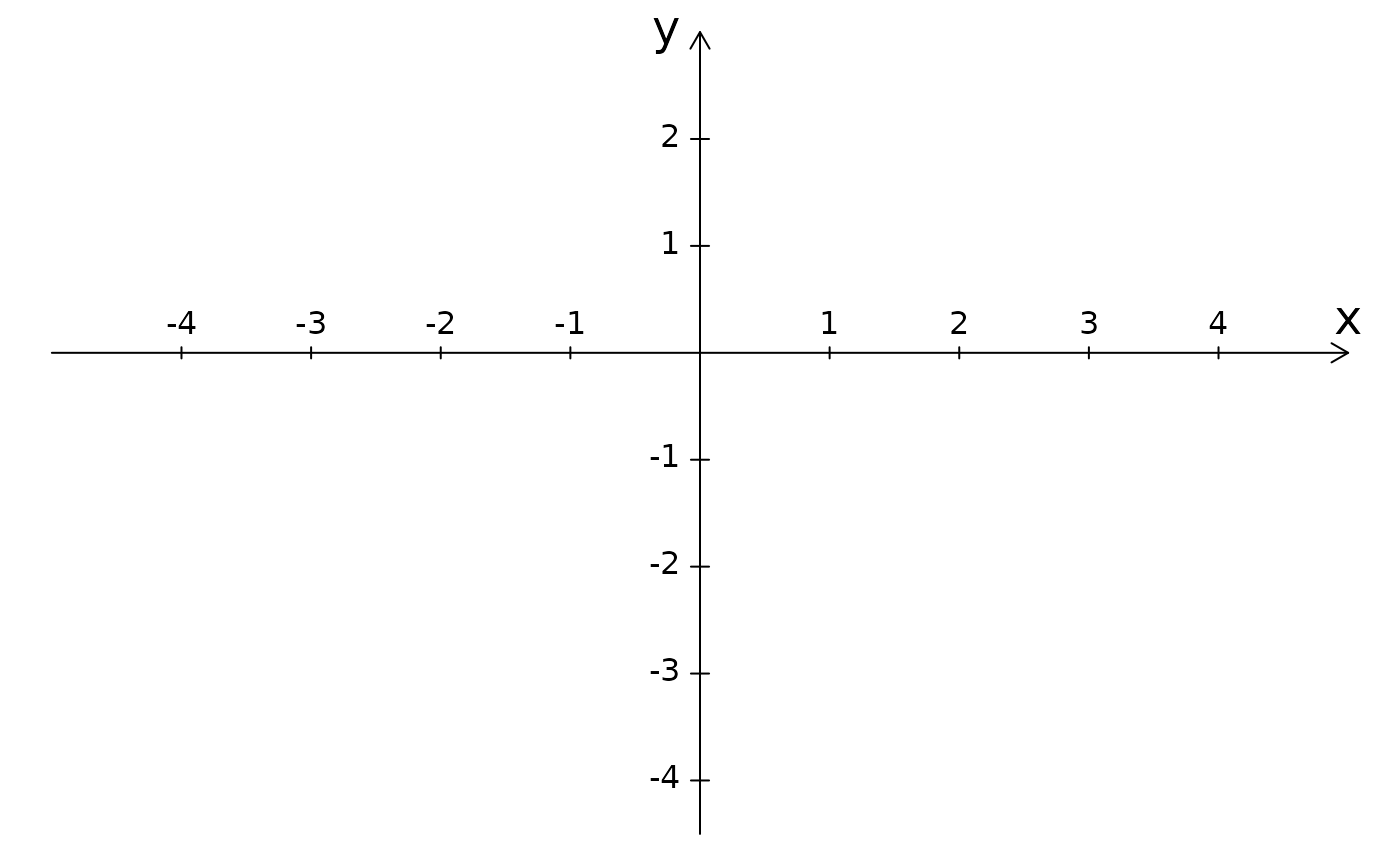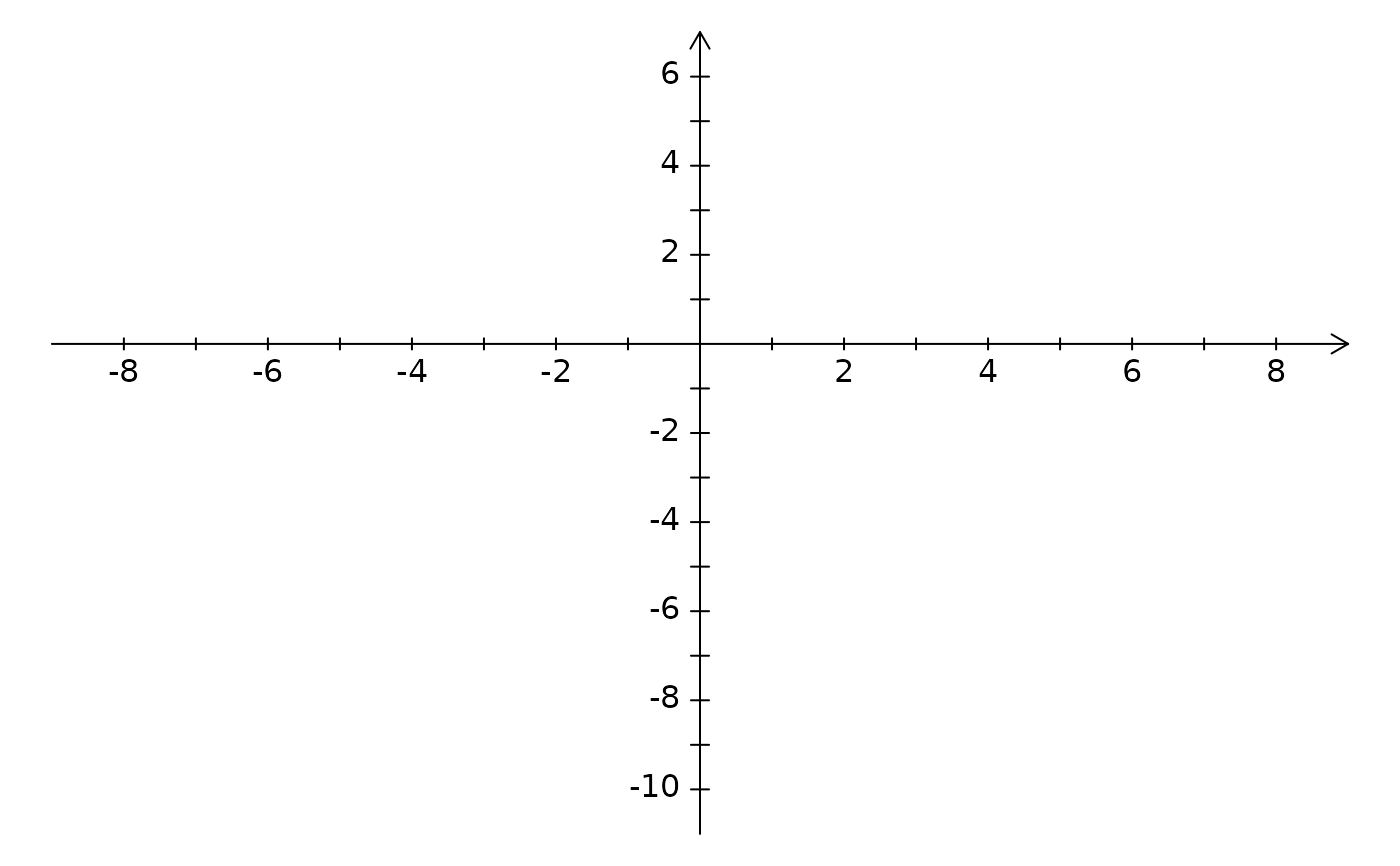Create a Cartesian Coordinate Plane.

## Usage

CCP(
xlim = c(-4, 4),
ylim = c(-4, 4),
mar = rep(0, 4),
length = 0.1,
tcl = 0.007,
xylab = FALSE,
ticks = 1,
ticklabs = 1,
xpos = 1,
ypos = 2,
cex.coord = 1,
cex.xylab = 1.5,
)

## Arguments

xlim

The x-limits for the plane (vector of length 2).

ylim

The y-limits for the plane (vector of length 2).

mar

Plotting margins.

length

The length argument is passed to the arrows function and is used to control the size of the arrow.

tcl

Tick size.

xylab

Whether x and y should be shown next to the labels.

ticks

How frequently tick marks should be shown on the axes. If a vector of length 2, the first argument will correspond to the x-axis and the second to the y-axis.

ticklabs

How frequently tick labels should be shown on the axes. If a vector of length 2, the first argument will correspond to the x-axis and the second to the y-axis.

xpos

The position of the labels on the x-axis. See the pos argument in the text function for additional details.

ypos

The position of the labels on the y-axis. See the pos argument in the text function for additional details.

cex.coord

Inflation factor for font size of the coordinates, where any value larger than zero is acceptable and 1 corresponds to the default.

cex.xylab

Inflation factor for font size of the x and y labels, where any value larger than zero is acceptable and 1 corresponds to the default.

Indicate whether a new plot should be created (FALSE, the default) or if the Cartesian Coordinate Plane should be added to the existing plot.

lsegments, dlsegments, ArrowLines

David Diez

## Examples


CCP()CCP(xylab = TRUE, ylim = c(-3.5, 2), xpos = 3, cex.coord = 1)CCP(xlim = c(-8, 8), ylim = c(-10, 6), ticklabs = c(2, 2), cex.xylab = 0.8)